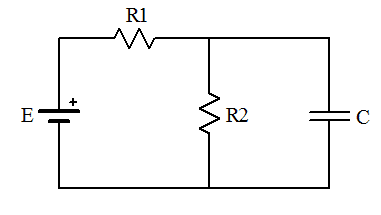# Maximum Charge on a Capacitor, Help

• Archived
Hi, thanks in advance to all who help

a) Determine the time constant for charging the capacitor in the circuit given.
b) what is the maximum charge on the capacitor?
R1
______vvvv____________
| | |
| < |
E R2 > C
| | |
|______________|______|

E = emf and C is the capacitor, sorry for the poor illustration.

Mentor's edit: Here's a better illustration:For a, I've determined the equivalent resistance of parallel resistors to be (1/R1 + 1/R2)^-1 = R1R2/(R1+R2), thus the time constant would be tau = CReq = CR1R2/(R1+R2)

For b) I have a feeling Qmax for the capacitor will be Qmax = CV where V is the voltage across R2 since R2 and C are parallel, but I can't figure out V across R2 in terms of E, R1, R2, C. Can anyone help? Thanks again.

Last edited by a moderator:

cnh1995
Homework Helper
Gold Member
For a, I've determined the equivalent resistance of parallel resistors to be (1/R1 + 1/R2)^-1 = R1R2/(R1+R2), thus the time constant would be tau = CReq = CR1R2/(R1+R2)
I got the same result after solving with differential equations.
Let the time constant be T.
Current supplied by the source is,
I(t)=ET/(R1R2C)*(1-e-t/T)+(E*e-t/T)/R1
From this equation, it can be verified that at t=0, I=E/R1(capacitor acts as a short at t=0) and at t=∞, I=E/(R1+R2)..(capacitor acts as open circuit at t=∞).
For b) I have a feeling Qmax for the capacitor will be Qmax = CV where V is the voltage across R2 since R2 and C are parallel, but I can't figure out V across R2 in terms of E, R1, R2, C.
In steady state, the capacitor will act as an open circuit. Hence, current will flow though the series combination of R1 and R2.
So, Voltage across C=Voltage across R2 in steady state= E*R2/(R1+R2)
Final charge on the capacitor=Q=CV= ECR2/(R1+R2).

•Ronie Bayron and gracy
cnh1995
Homework Helper
Gold Member
The above approach involves some mathematical calculations and a generalized expression for current I(t). Instead, I believe the best and simplest approach to solve these two questions a and b is Thevenin's theorem.

•gracy
Hi, thanks in advance to all who help

a) Determine the time constant for charging the capacitor in the circuit given.
b) what is the maximum charge on the capacitor?
R1
______vvvv____________
| | |
| < |
E R2 > C
| | |
|______________|______|

E = emf and C is the capacitor, sorry for the poor illustration.

Mentor's edit: Here's a better illustration:
View attachment 95530

For a, I've determined the equivalent resistance of parallel resistors to be (1/R1 + 1/R2)^-1 = R1R2/(R1+R2), thus the time constant would be tau = CReq = CR1R2/(R1+R2)

For b) I have a feeling Qmax for the capacitor will be Qmax = CV where V is the voltage across R2 since R2 and C are parallel, but I can't figure out V across R2 in terms of E, R1, R2, C. Can anyone help? Thanks again.

Why do you regard R1 and R2 as being in parallel?
When C is fully charged then the current through the capacitor is ?

Which makes the fial voltage across C ? ?

Hence the max charge will be ? ? ?

cnh1995
Homework Helper
Gold Member
Why do you regard R1 and R2 as being in parallel?
When C is fully charged then the current through the capacitor is ?

Which makes the final voltage across C ? ?

Hence the max charge will be ? ? ?
Are those your own queries or are you trying to give hints to the OP?

Hi, thanks in advance to all who help

a) Determine the time constant for charging the capacitor in the circuit given.
b) what is the maximum charge on the capacitor?
R1
______vvvv____________
| | |
| < |
E R2 > C
| | |
|______________|______|

E = emf and C is the capacitor, sorry for the poor illustration.

Mentor's edit: Here's a better illustration:
View attachment 95530

For a, I've determined the equivalent resistance of parallel resistors to be (1/R1 + 1/R2)^-1 = R1R2/(R1+R2), thus the time constant would be tau = CReq = CR1R2/(R1+R2)

For b) I have a feeling Qmax for the capacitor will be Qmax = CV where V is the voltage across R2 since R2 and C are parallel, but I can't figure out V across R2 in terms of E, R1, R2, C. Can anyone help? Thanks again.
Vc=VR2 where R1 & R2 are voltage divider.
I = E/(R1+R2) and VR2=IR2, so Vc =E[R2/(R1+R2)]

Vc=VR2 where R1 & R2 are voltage divider.
I = E/(R1+R2) and VR2=IR2, so Vc =E[R2/(R1+R2)]
Since C=Q/Vc, Q =C Vc, so the charge Q depends on the value of capacitance you put on your circuit and magnitude of Vc